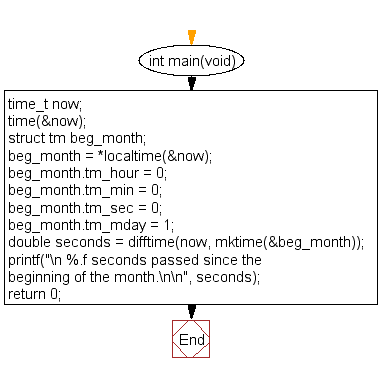﻿ C : Number of seconds since the beginning of the month# C Exercises: Compute the number of seconds passed since the beginning of the month

## C Date Time: Exercise-2 with Solution

Write a program in C to compute the number of seconds passed since the beginning of the month.

Sample Solution:

C Code:

``````#include <stdio.h>
#include <time.h>

int main(void)
{
time_t now;
time(&now);

struct tm beg_month;
beg_month = *localtime(&now);
beg_month.tm_hour = 0;
beg_month.tm_min = 0;
beg_month.tm_sec = 0;
beg_month.tm_mday = 1;

double seconds = difftime(now, mktime(&beg_month));
printf("\n %.f seconds passed since the beginning of the month.\n\n", seconds);
return 0;
}
```
```

Sample Output:

``` 222084 seconds passed since the beginning of the month.
```

N.B.: The result may varry for your current system date and time.

Flowchart:C Programming Code Editor:

What is the difficulty level of this exercise?

Test your Programming skills with w3resource's quiz.

﻿

## C Programming: Tips of the Day

How I can print to stderr in C?

The syntax is almost the same as printf. With printf you give the string format and its contents i.e.:

`printf("my %s has %d chars\n", "string format", 30);`

With fprintf it is the same, except now you are also specifying the place to print to:

```File *myFile;
...
fprintf( myFile, "my %s has %d chars\n", "string format", 30);```

`fprintf( stderr, "my %s has %d chars\n", "string format", 30);`